# Is Mean Temp in 2 phase Heat Exchangers Higher Than Logarithmic Mean?

• B
Chestermiller
Mentor
Would it be accurate to say if phase change occurs in none of the 3 options... at 7MPa, the temp drops from 125C to 50C, and at 5MPa, the temp drops from 125C to 30C, and the 2.5MPa case, the temp drops from 125C to 0C, none of these should have left the gas phase during these temperature swings, and Temp vs KJ/KG line for all of those approximates a straight line. But in the 7MPa and 5MPa cases, if we continue to decrease the temperature, the temperature stays higher for longer for the same amount of energy extracted than would be the case if the logarithmic trend continued, would they not?

View attachment 286318
No exactly. The LMTD and analyses like the present one are typically used to determine the required heat transfer surface area A to bring about the desired amount of heat transfer. The temperature difference between the CO2 and the hot working fluid provides the driving force for the heat exchange, and the lower this temperature difference is, the more area is required. If insufficient heat transfer surface area is provided, the desired amount of heat transfer will not occur (assuming that the overall heat transfer coefficient U is constant). If the cases at 5 MPa and 7 MPa are being designed using the LMTD to determine the heat transfer area, because the LMTD in these cases overestimates the heat transfer driving force, the required heat transfer area will be incorrectly underestimated. A system designed on this basis would not transfer sufficient heat to the working fluid.

No exactly. The LMTD and analyses like the present one are typically used to determine the required heat transfer surface area A to bring about the desired amount of heat transfer. The temperature difference between the CO2 and the hot working fluid provides the driving force for the heat exchange, and the lower this temperature difference is, the more area is required. If insufficient heat transfer surface area is provided, the desired amount of heat transfer will not occur (assuming that the overall heat transfer coefficient U is constant). If the cases at 5 MPa and 7 MPa are being designed using the LMTD to determine the heat transfer area, because the LMTD in these cases overestimates the heat transfer driving force, the required heat transfer area will be incorrectly underestimated. A system designed on this basis would not transfer sufficient heat to the working fluid.

I'm now very confused. Is the below pictogram wrong?

The bottom picture (phase change gas-> liquid) shows a higher average temperature when it reaches equilibrium than the top picture (no phase change). Both have the same starting temperatures. Wouldn't the 7MPa option be more like the bottom picture (higher average temp at equilibrium with the same starting temps on account of phase change latent heat), and the 2.5MPa option more like the top picture (lower average temp at equilibrium with the same starting temps on account of no phase change latent heat)https://www.swepusa.com/refrigerant-handbook/1.-basic-heat-transfer/as5/

Chestermiller
Mentor
I'm now very confused. Is the below pictogram wrong?

The bottom picture (phase change gas-> liquid) shows a higher average temperature when it reaches equilibrium than the top picture (no phase change). Both have the same starting temperatures. Wouldn't the 7MPa option be more like the bottom picture (higher average temp at equilibrium with the same starting temps on account of phase change latent heat), and the 2.5MPa option more like the top picture (lower average temp at equilibrium with the same starting temps on account of no phase change latent heat)https://www.swepusa.com/refrigerant-handbook/1.-basic-heat-transfer/as5/
We are not comparing the different pressure cases with one another. We are comparing each of them with the hypothetical temperature vs enthalpy relationship that would have to exist for the CO2 in order for the LMTD to apply. In all three cases, the hypothetical (leading to the LMTD) average temperature of the CO2 profile for heat transfer driving force would be about 60 C, and this would also be the effective average temperature of the CO2 for heat transfer in the 2.5 MPa case. The effective average temperature of the CO2 in the actual 7 MPa case and in the 5 MPa case would be substantially lower than 60 C. In the 7 MPa case, from the graph (by eyeball), I"m guessing an effective average temperature of about 50 C and, in the 5 MPa case, a value of about 40 C.

If I have 1 kg of CO2 at 127C at 2.5MPa, and it's -10C outside, and I let it cool to 0C at constant pressure (by convection to the environment), it has lost 140kJ.

If I have 1kg of CO2 at 127C at 7MPa, and it's -10C outside, and I let it naturally cool by convection so that the same 140kJ exits the 1kg at constant pressure, it's still 35C...

...that's 35C warmer than the 2.5MPa CO2 that has lost the same energy to the environment.

Wouldn't that mean the average temperature is higher with the 7MPa CO2 than the 2.5MPa CO2, because after releasing the same energy, the 7MPa C02 is warmer?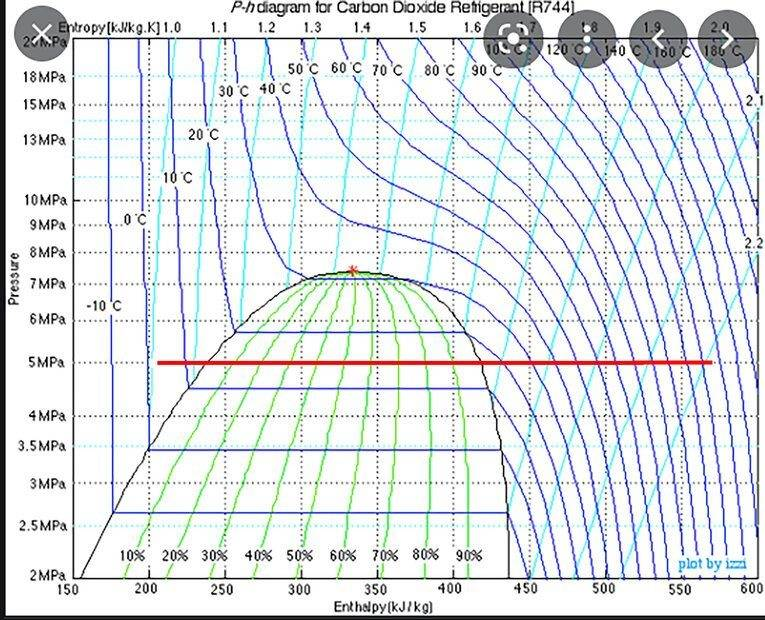Chestermiller
Mentor
The comparison should made be for the same temperature change. In that case, for the 7MPa , a larger fraction of the heat is transferred at lower temperatures.

Last edited:
The comparison should made be for the same temperature change.
ok so:

1kg, 7MPa, 127C to 0C goes from 560kJ/kg to 200kJ/kg at constant pressure so loses 360kJ to the -10C environment.

1kg, 2.5MPa, 127C to 0C goes from 590kJ/kg to 450kJ/kg at constant pressure so loses 140kJ to the -10C environment.

Since the environment acquires 220kJ more with the 7MPa than the 2.5MPa for the same temperature change, doesn't that make the 7MPa, warmer on average, and wouldn't it mean the 2.5MPa loses it's temperature according to the logarithmic formula since it has no phase change (stays as a gas) while the 7MPa converts from a gas to a liquid?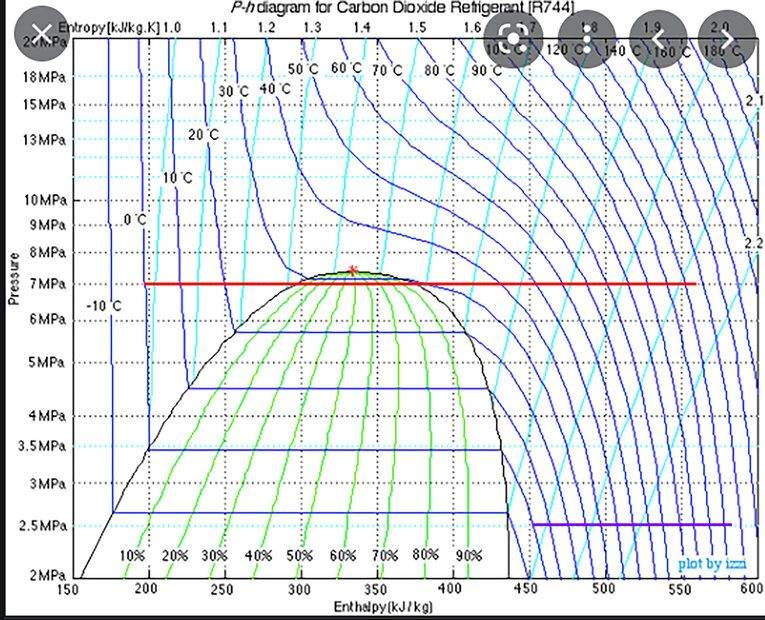Chestermiller
Mentor
The 2.5 would definitely follow the log formula. The 7.5 would transfer more heat with a lower average temperature difference (relative to the -10 C), and would thus require moch more heat transfer area A.

If the -10C environment is sealed and insulated, and the 2.5MPa 1kg CO2 drops from 127C to 0C (remaining a gas), and at that point the experiment is reset and repeated with the 7MPa 1kg from 127C to 0C, by the time the environment has warmed to the same temperature as the end of the first experiment, the 7MPa 1kg is still 35C. Wouldn't a thermometer measure the cube 35C warmer at that point in the experiment? If the cube is measured warmer, won't heat transfer still be occurring at a higher rate than the first experiment? If that's all true how could could the average temperature of the 7MPa 1kg as tabulated at any point in the experiment be lower?

Chestermiller
Mentor
I’m obviously having a hard time explaining what I mean. I think I’ll stop for now and give others a chance to respond.

I really do appreciate the help you've given and I feel my understanding has advanced thanks to you but still not fully grasping it.

After 2, same weight, compressed samples of C02 at constant pressure transfer the same amount of energy to sub-zero C environments in separate experiments (one at 5MPa and the other at 2.5MPa, both starting at 125C), at the end of the experiments, the 5MPa CO2 sample is 25C warmer than the 2.5MPa CO2 sample... the 2.5MPa sample follows the log mean formula so wouldn't the 5MPa sample be warmer than the log mean formula implies, on average?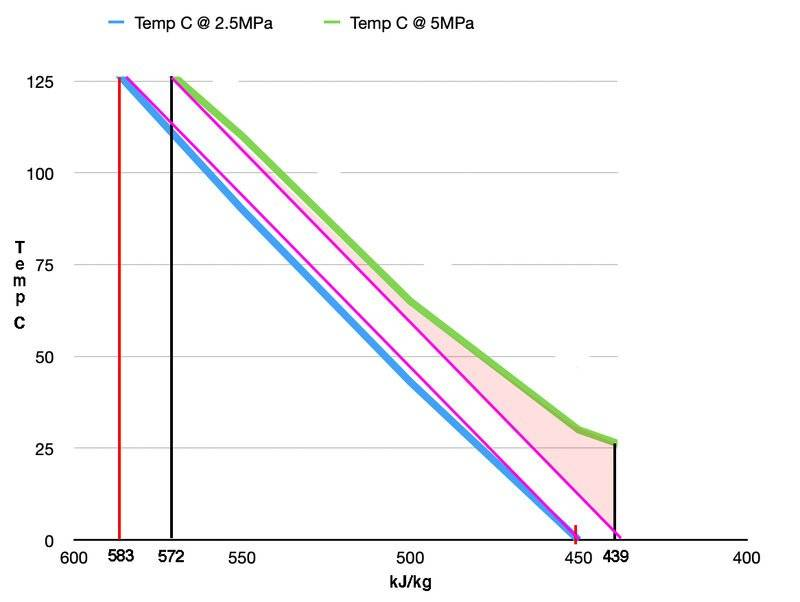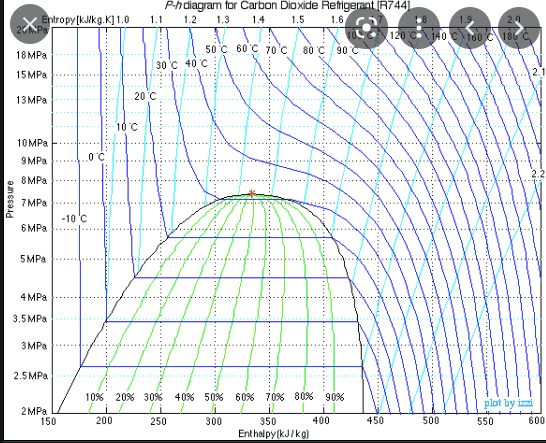Last edited:
Chestermiller
Mentor
The 5 MPa would not be higher than its own log-mean formula (which would correspond to a straight line drawn from tip to toe of the green line).

The 5 MPa would not be higher than its own log-mean formula (which would correspond to a straight line drawn from tip to toe of the green line).

If the hot side CO2 refrigerant of a heat pump is 2.5MPa from 125C to 0C, I thought the average temperature of the hot heat exchanger can be calculated by the log mean formula, and its phase stays a gas.

But if we have 1kg samples of CO2 at fixed pressure 2.5MPa and 5MPa starting at 125C, and we extract the same 133kJ energy from the 5MPa and 2.5MPa samples to a cold sub-zero C environment at constant CO2 pressure, the 5MPa temperature is 25C warmer at the end than the 0C 2.5MPa temperature.

Chestermiller
Mentor
If the hot side CO2 refrigerant of a heat pump is 2.5MPa from 125C to 0C, I thought the average temperature of the hot heat exchanger can be calculated by the log mean formula, and its phase stays a gas.

But if we have 1kg samples of CO2 at fixed pressure 2.5MPa and 5MPa starting at 125C, and we extract the same 133kJ energy from the 5MPa and 2.5MPa samples to a cold sub-zero C environment at constant CO2 pressure, the 5MPa temperature is 25C warmer at the end than the 0C 2.5MPa temperature.
All this is telling us is that, for the same amount of heat transferred, the 5 MPa case requires less heat transfer area.

Last edited:
The heat capacities are different along the curves for the two different pressure cases.

So the Lorenz COP of the heat pump with ~125C (126.85C) to ~0C (1.85C) on the hot side is 3.95... That's assuming -48.15C coming out of the expansion valve heated to 1.85C by the ocean water.

Compared to this case:
"If the hot side CO2 refrigerant of a heat pump is 2.5MPa from 125C to 0C, I thought the average temperature of the hot heat exchanger can be calculated by the log mean formula, and its phase stays a gas."

...but with the same mass flow rate, since the 5MPa CO2 has higher heat capacity per kg than the 2.5MPa CO2, couldn't we hook one stirling up to the part of the 5MPa hot side of the heat exchanger that goes from 125C to 25C, and get the same amount of energy out or slightly more because of slightly higher efficiency because the same amount of energy comes out of the the coolant from 125C to 25C at 5MPa as with 125C to 0C at 2.5MPa, but the avg temp of 125C and 25C is hotter than 125C and 0C, so the higher average temp would make that stirling more efficient, because the hot side avg temperature is hotter from 125C to 25C than 125C to 0C, then add a second stirling to the rest of the 5MPa hot side heat exchanger from 25C to 0C (cold side stirlings connected to cold side heat pump)... wouldn't that stirling be less efficient because the the average temp on its hot side is lower, but it doesn't matter because the 125C to 25C hot side stirling was putting out as much if not more power as the single 125C to 0C 2.5MPA hot side stirling on account of the higher heat capacity and higher average hot temp?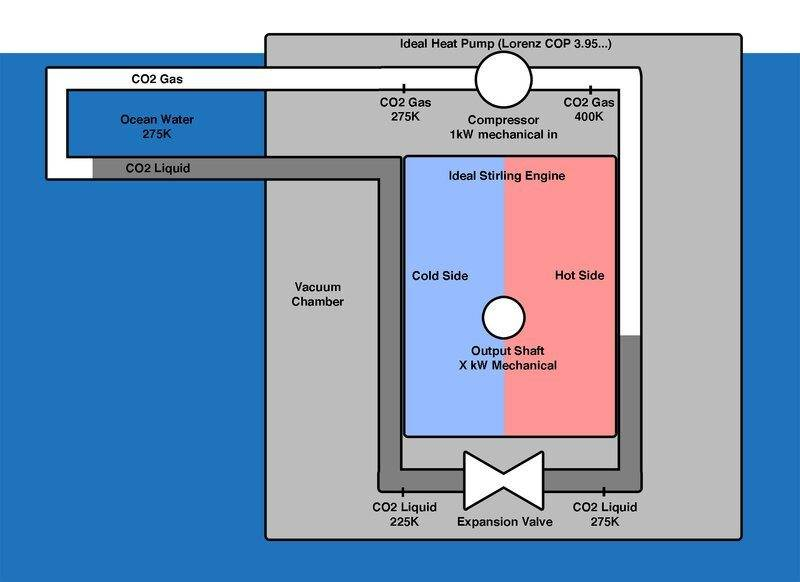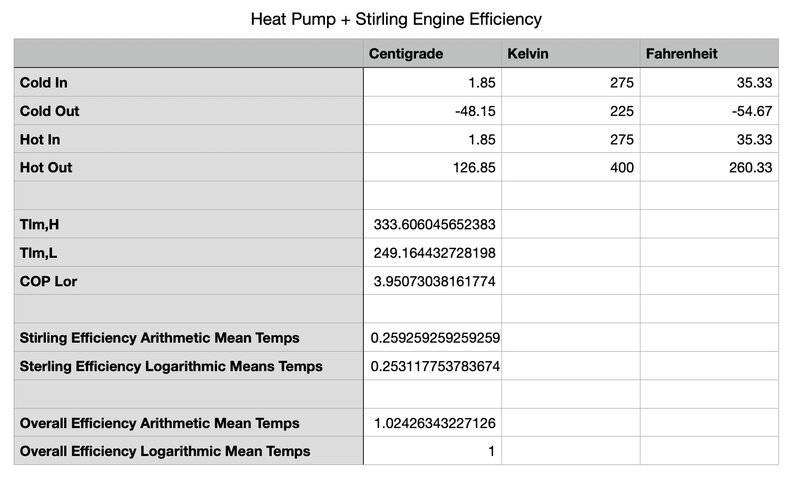Last edited:
Chestermiller
Mentor
Did you read what I said about the different heat transfer areas required for the same heat load?

Chestermiller
Mentor
So the Lorenz COP of the heat pump with ~125C (126.85C) to ~0C (1.85C) on the hot side is 3.95... That's assuming -48.15C coming out of the expansion valve heated to 1.85C by the ocean water.

Compared to this case:
"If the hot side CO2 refrigerant of a heat pump is 2.5MPa from 125C to 0C, I thought the average temperature of the hot heat exchanger can be calculated by the log mean formula, and its phase stays a gas."

...but with the same mass flow rate, since the 5MPa CO2 has higher heat capacity per kg than the 2.5MPa CO2, couldn't we hook one stirling up to the part of the 5MPa hot side of the heat exchanger that goes from 125C to 25C, and get the same amount of energy out or slightly more because of slightly higher efficiency because the same amount of energy comes out of the the coolant from 125C to 25C at 5MPa as with 125C to 0C at 2.5MPa, but the avg temp of 125C and 25C is hotter than 125C and 0C, so the higher average temp would make that stirling more efficient, because the hot side avg temperature is hotter from 125C to 25C than 125C to 0C, then add a second stirling to the rest of the 5MPa hot side heat exchanger from 25C to 0C (cold side stirlings connected to cold side heat pump)... wouldn't that stirling be less efficient because the the average temp on its hot side is lower, but it doesn't matter because the 125C to 25C hot side stirling was putting out as much if not more power as the single 125C to 0C 2.5MPA hot side stirling on account of the higher heat capacity and higher average hot temp?

View attachment 286339

View attachment 286340
Sorry, this is too complicated. Too much for me to deal with.

Sorry, this is too complicated. Too much for me to deal with.

This is what I meant:

2.5MPa 125C (400K) to 0C (275K) hot side (all gas) - avg temp of hot side is the logarithmic mean temp: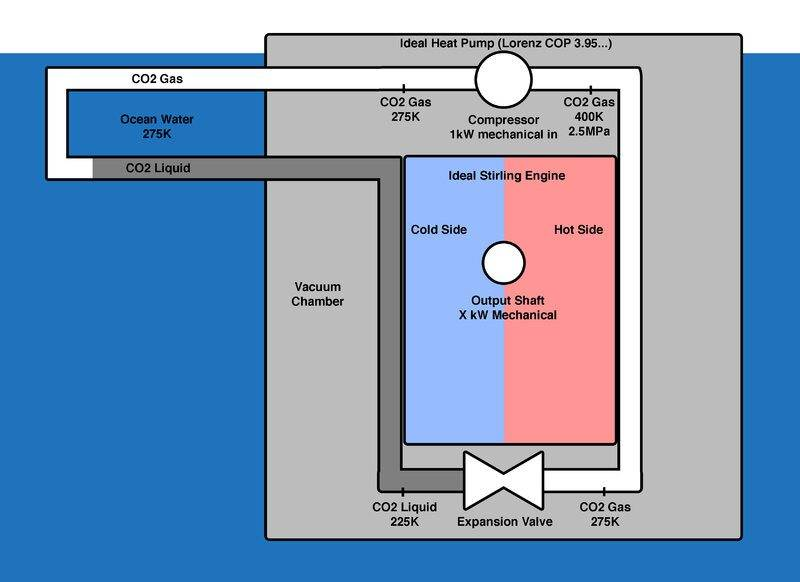5MPa 125C to 0C hot side (gas to liquid):

For the same mass flow rate gas loses the same amount of heat energy from 125C (400K) to 25C (298K) as the 1st case (from 125C to 0C) through the upper stirling, but the average temp between 125C and 25C is higher than the 1st case of 125C to 0C, which makes the upper stirling more efficient while transferring the same amount of heat as the single stirling in the 1st case, so it should make as much power if not more as compared to the 1st case. The lower stirling then generates additional power from the remaining 25C (298K) to 0C (275K) on the hot side.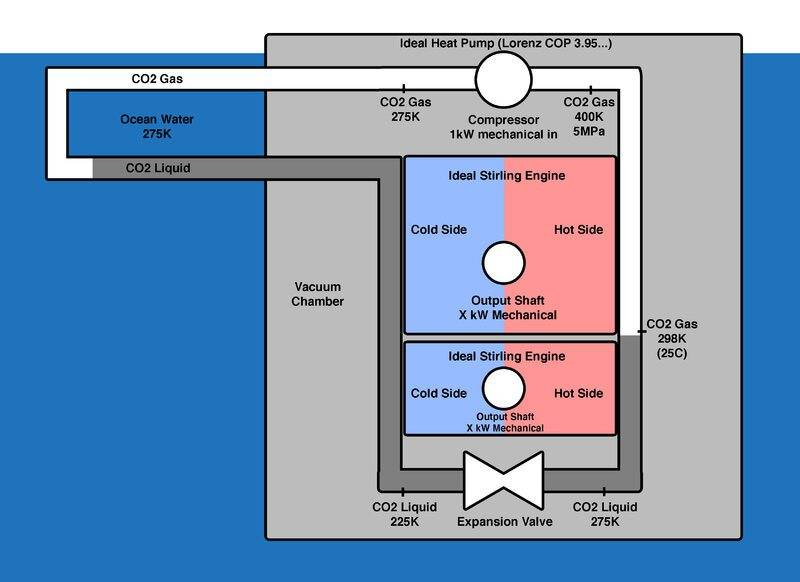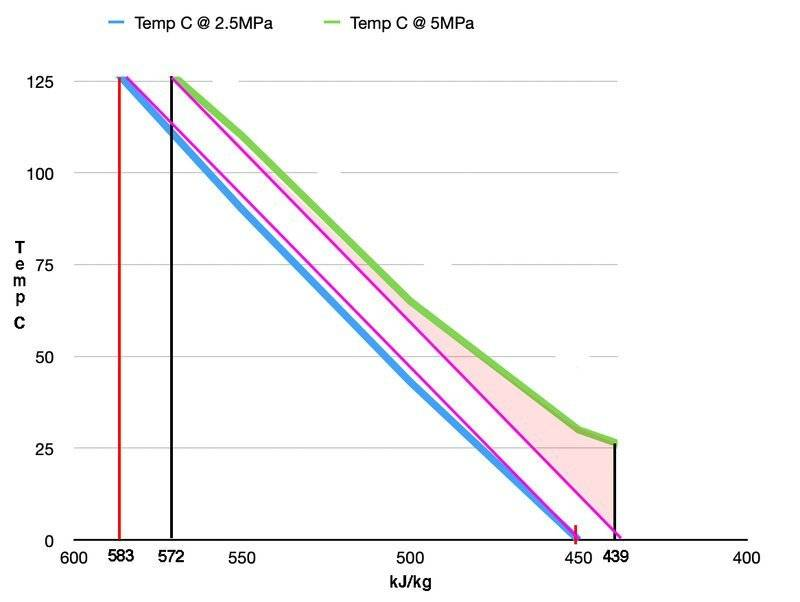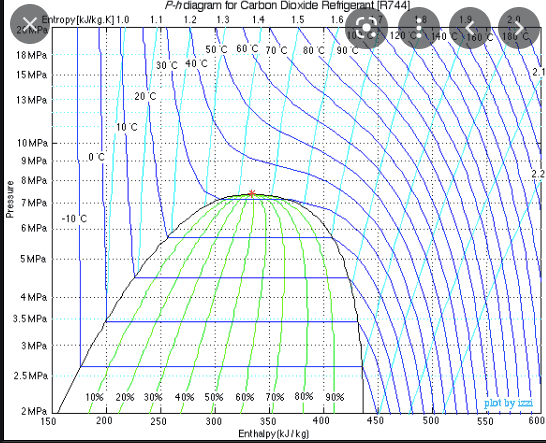Last edited:
Chestermiller
Mentor
Let's take a step back to post #36. For the same amount of heat transferred, the temperature decrease for the 2.5 MPa is larger than for the 5 MPa, and the average temperature for the 2.5 MPa is less than the 5 MPa. This means that there is a larger temperature driving force for the 5 MPa than for the 2.5 MPa. For the same heat transferred, mass flow rate, and overall heat transfer coefficient, this tells us that more heat transfer area will be required for the 2.5 MPa cased than for the 5 MPa.

Regarding the comparisons between the LMTD and the actual effective temperature driving force, the LMTD will be about equal to effective temperature driving force for the 2.5 MPa case; but, for the 5 MPa case, the LMTD (based on the initial and final temperature for this case) will be less than the effective temperature driving force for this case.

Chestermiller
Mentor
ok so:

1kg, 7MPa, 127C to 0C goes from 560kJ/kg to 200kJ/kg at constant pressure so loses 360kJ to the -10C environment.

1kg, 2.5MPa, 127C to 0C goes from 590kJ/kg to 450kJ/kg at constant pressure so loses 140kJ to the -10C environment.

Since the environment acquires 220kJ more with the 7MPa than the 2.5MPa for the same temperature change, doesn't that make the 7MPa, warmer on average, and wouldn't it mean the 2.5MPa loses it's temperature according to the logarithmic formula since it has no phase change (stays as a gas) while the 7MPa converts from a gas to a liquid?

View attachment 286330
For the same mass flow rate, more heat is transferred in the 7 MPa case than in the 2.5 MPa case, even though the average temperature on the CO2 side of the heat transfer surface is lower for the 7MPa case than the 2.5 MPa case. This is an indication that more heat transfer area will be required for the 7 MPa case both because of the lower heat transfer driving force and because of the larger amount of heat transferred.

Let's take a step back to post #36. For the same amount of heat transferred, the temperature decrease for the 2.5 MPa is larger than for the 5 MPa, and the average temperature for the 2.5 MPa is less than the 5 MPa. This means that there is a larger temperature driving force for the 5 MPa than for the 2.5 MPa. For the same heat transferred, mass flow rate, and overall heat transfer coefficient, this tells us that more heat transfer area will be required for the 2.5 MPa cased than for the 5 MPa.

Regarding the comparisons between the LMTD and the actual effective temperature driving force, the LMTD will be about equal to effective temperature driving force for the 2.5 MPa case; but, for the 5 MPa case, the LMTD (based on the initial and final temperature for this case) will be less than the effective temperature driving force for this case.
In this picture (5MPa), when the C02 cools from 125C (400K) to 25C (298K), it’s still a gas, but has released the same energy as the 2.5MPa case from 125C all the way to 0C (275k).

Since the same energy has transferred from 125C to 25C & no phase change occurs, if I take the logarithmic mean temperature of 125C and 25C, that’s higher than the logarithmic mean of 125C and 0C.

A higher logarithmic mean means higher stirling efficiency, but the same heat energy transferred for the same mass flow rate, so the upper stirling should produce more mechanical power than the only stirling in in the 2.5MPa case. But the gas has only cooled to 25C, and we can go all the way down to ~0C with the setup, so I added a second stirling.

The 2.5MPa setup already gets 100% mechanical power out as mechanical power in if you multiply the Lorenz COP by the stirling efficiency for the logarithmic mean temps of both sides of that setup.Last edited:
Chestermiller
Mentor
I'm somewhat confused. Can you please explain to me how this device operates, including how the Stirling Engine comes in, and what its working fluid is? It looks to me like the CO2 is going through a standard vapor compression refrigeration cycle.

•russ_watters
I'm somewhat confused. Can you please explain to me how this device operates, including how the Stirling Engine comes in, and what its working fluid is? It looks to me like the CO2 is going through a standard vapor compression refrigeration cycle.

I used equations 2, 3, and 4 in this paper to calculate the heat pump lorenz COP:

https://backend.orbit.dtu.dk/ws/portalfiles/portal/149827036/Contribution_1380_final.pdf

If you calculate the log mean temps of both sides, and use equation 1 to calculate the stirling efficiency, when you multiply the stirling efficiency by the lorenz cop, it’s always exactly 100% as far as I can tell.

My understanding is that describes the 2.5MPa setup with no phase changing heat exchangers.

Chestermiller
Mentor
I used equations 2, 3, and 4 in this paper to calculate the heat pump lorenz COP:

https://backend.orbit.dtu.dk/ws/portalfiles/portal/149827036/Contribution_1380_final.pdf

If you calculate the log mean temps of both sides, and use equation 1 to calculate the stirling efficiency, when you multiply the stirling efficiency by the lorenz cop, it’s always exactly 100% as far as I can tell.

My understanding is that describes the 2.5MPa setup with no phase changing heat exchangers.
I need something much more basic than this. I need you to describe in words the operation of the exact system the you are using here.

russ_watters
Mentor
I think there's a design issue in the diagram and still a conceptual issue with the entire exercise:

The Carnot efficiency is based on the reservoir temperatures. Lorentz efficiency is based on the working fluid temperatures and is necessarily worse than the Carnot efficiency. Always keep that in mind, because if you are getting a heat pump and Stirling engine efficiency that are exact inverses via the Lorenz efficiency, something is wrong: the Stirling efficiency should be worse. But if you're going through all this effort to define the cycles, it seems to me like you might as well calculate a more "real" efficiency using the heat transfer. If you have the starting and end states you can just calculate the heat transfer.

The cycle you drew is missing the heat rejection from the Stirling engine. CO2 liquid comes in at 225C -- so then what temperature does it leave at? I didn't go back through and look for where you did the calc, but the fact that it doesn't appear in the diagram implies to me that you used 225C as the cold side reservoir, which is the wrong temperature (too cold), giving you way too high of an efficiency on the Stirling engine.

One of the other problems with just using the temperatures is that it can hide another flaw in the cycle: if the heat transfers don't match then you also have a problem with the flow rates not matching. One of the problems with Stirling engines is because of the low delta-t's, you need huge flow rates and heat exchangers to capture a useful amount of heat.

Er...wait a minute, why do we even have the water in the cycle? There's a heat leak here. The heats won't sum to zero.

Last edited:
•Chestermiller